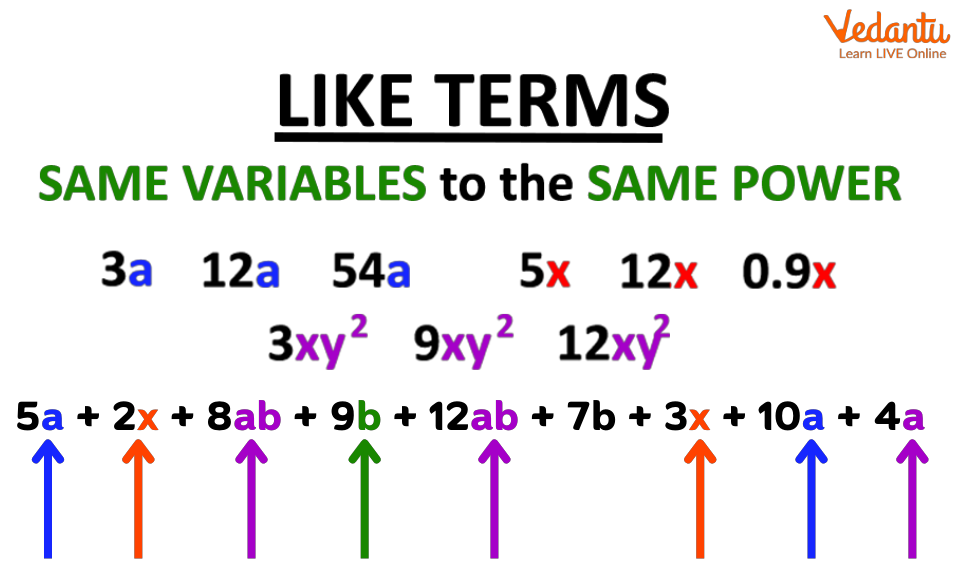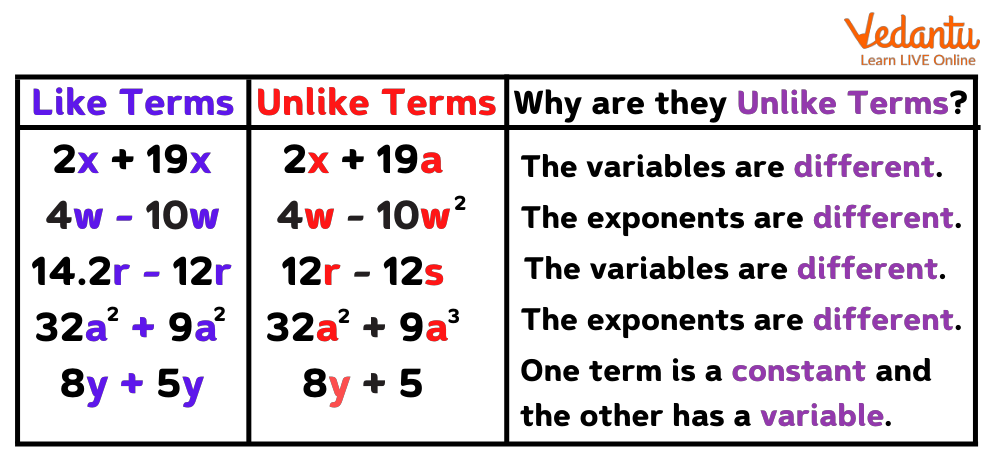Courses
Courses for Kids
Free study material
Free LIVE classes
More

# Term - What is it?LIVE
Join Vedantu’s FREE Mastercalss

## What is a Term in Mathematics?

A term in mathematics is nothing but a single expression. The term can be a single number - either positive or negative, or it can be a variable, or it can be more than one variable multiplied together. The variables are often written with numbers in front of them and these numbers are called coefficients. Let’s have a look at a few examples:

Single number as a term: 15

A variable: a

More than one variable: 2x3y24z3

In algebra, two or more terms are often joined to make an expression. For example:

2a + 3b

4c - 5d2

6x + 7y2 - 8z3

4x2y + 5a2b3 - 6m3n4

There are two types of terms: Like terms and unlike terms. Let’s learn about them.

## Like Terms

Like terms are the terms whose variables are similar. For example - 2x2 and 3x2 are like terms because the variable x2 is the same. A few more examples are: 3a3, 5a3, and 7a3; 2x2y, 3x2y, and 4x2yLike Terms

To solve the algebraic expressions, we need to combine the like terms and simplify the expressions. For example:

3x2 + 2x + 9 - 2x2+ 5x - 3

= 3x2 - 2x2 + 2x + 5x + 9 - 3

= x2 + 7x + 6

Would you like to solve an exercise?

## Exercise on Like Terms

Here are a few questions:

1. 2r2+1+(−4r2)+7

2. 4x + 2y + 3x - y

3. -5m + (-6n) + 3m - 2n

4. 3a2 + 5b + 6a + a2 - 2b

5. -z2 + 12z2 - 5z + 8z3 - 15z

Solutions:

1. 2r2+1+(−4r2)+7

= 2r2 - 4r2 + 1 + 7

=-2r2 + 8

1. 4x + 2y + 3x - y

= 4x + 3x + 2y - y

= 7x + y

1. -5m + (-6n) + 3m - 2n

= -5m + 3m - 6n - 2n

=2m - 8n

1. 3a2 + 5b + 6a + a2 - 2b

= 3a2 + a26a + 5b - 2b

= 4a2 + 6a + 3b

1. -z2 + 12z2 - 5z + 8z3 - 15z

= 8z3 - z2 + 12z25z - 15z

8z3 + 11z2 - 20z

## Unlike Terms

Unlike terms are the terms whose variables are different from each other. Example:

4x and 9y; 3x2 and 5y; 4a2 + 5b3 - 6z4

Simplifying expressions with like and unlike terms:

2ab2 - 7c2 + 3abc - 4ab2 + 8c2 - ab

= 2ab2 - 4ab2 - 7c2 + 8c2 + 3abc - ab

= -2ab2 + c2 + 3abc - abLike and Unlike terms

## What Have We Observed?

So what have we observed? We have observed that while simplifying any expression, only the like terms are added or subtracted. The, unlike terms, remain as they are in an expression.

Did you enjoy learning about the like and unlike terms? We have other interesting math ‘magic’ topics as well for you to explore. You can explore it through our website and also download the app from the play store.

Last updated date: 21st Sep 2023
Total views: 137.1k
Views today: 2.37k

## FAQs on Term - What is it?

1. How many terms are there in an expression?

One or more terms can make up an algebraic expression. A constant, a variable, a product of more than two variables (xy), or a product of a variable and a constant can all be used as terms in an expression. The terms, when added together, form an expression.

2. What is a coefficient?

In an algebraic equation, a coefficient represents the numerical factor of a term made up of constants and variables. The terms' coefficients can be either positive or negative. It's possible that they're fractional in nature.

3. What is a variable?

Variables are terms made up of undefined values that, when substituted with different integers, can take on different integer values. A variable term can be made up of one or more variables, which can be the same or different.